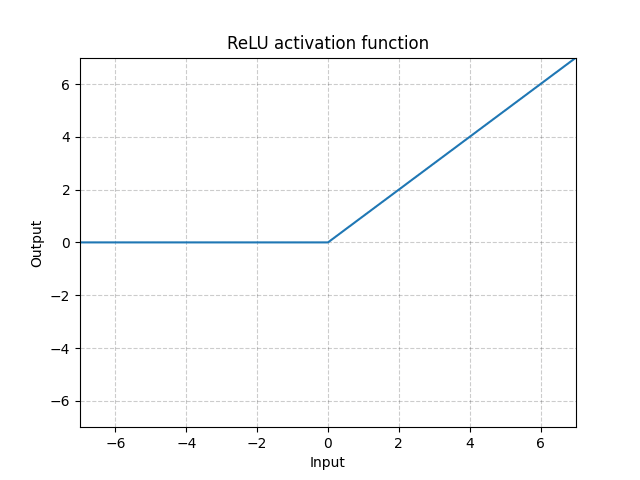/PyTorch

# ReLU

class torch.nn.ReLU(inplace: bool = False) [source]

Applies the rectified linear unit function element-wise:

$\text{ReLU}(x) = (x)^+ = \max(0, x)$

Parameters

inplace – can optionally do the operation in-place. Default: False

Shape:
• Input: $(N, *)$ where * means, any number of additional dimensions
• Output: $(N, *)$ , same shape as the inputExamples:

  >>> m = nn.ReLU()
>>> input = torch.randn(2)
>>> output = m(input)

An implementation of CReLU - https://arxiv.org/abs/1603.05201

>>> m = nn.ReLU()
>>> input = torch.randn(2).unsqueeze(0)
>>> output = torch.cat((m(input),m(-input)))


© 2019 Torch Contributors
Licensed under the 3-clause BSD License.
https://pytorch.org/docs/1.7.0/generated/torch.nn.ReLU.html